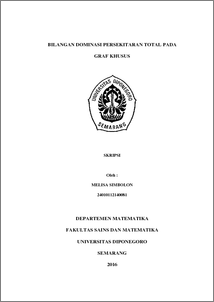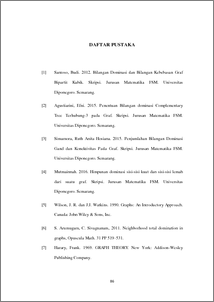# BILANGAN DOMINASI PERSEKITARAN TOTAL PADA GRAF KHUSUS

SIMBOLON, MELISA (2016) BILANGAN DOMINASI PERSEKITARAN TOTAL PADA GRAF KHUSUS. Undergraduate thesis, UNDIP.Preview
PDF
621KbPDF
Restricted to Repository staff only

4MbPreview
PDF
129Kb

## Abstract

In this paper is discussed about exact value of neighbourhood total domination number in graphs. Let graph . A set of be a subset of is called a dominating set if each vertex of is adjacent to at least one vertex of is graph . The minimum cardinality of dominating set in is called domination number and denoted . Let be a subset of , set is called a neighbourhood set if ⋃ 〈 〉 with 〈 〉 the induced subgraph of by . A dominating set of a graph is called neighbourhood total dominating set (ntdset) if the induced subgraph 〈 〉 contains no isolated vertices. The minimum cardinality of a ntd-set of is called the neighbourhood total domination number of and is denoted by . Further be obtained the exact value in path graphs, cycle graphs, tree graphs, star graphs, complete bipartite graphs, wheel graphs, ladder graphs, m graphs, and s graphs. Keywords : Dominating set, domination number, neighbourhood set, neighbourhood total domination set, neighbourhood total domination number.

Item Type: Thesis (Undergraduate) Q Science > QA Mathematics 84277 INVALID USER 14 Jun 2022 08:53 14 Jun 2022 08:53

Repository Staff Only: item control page